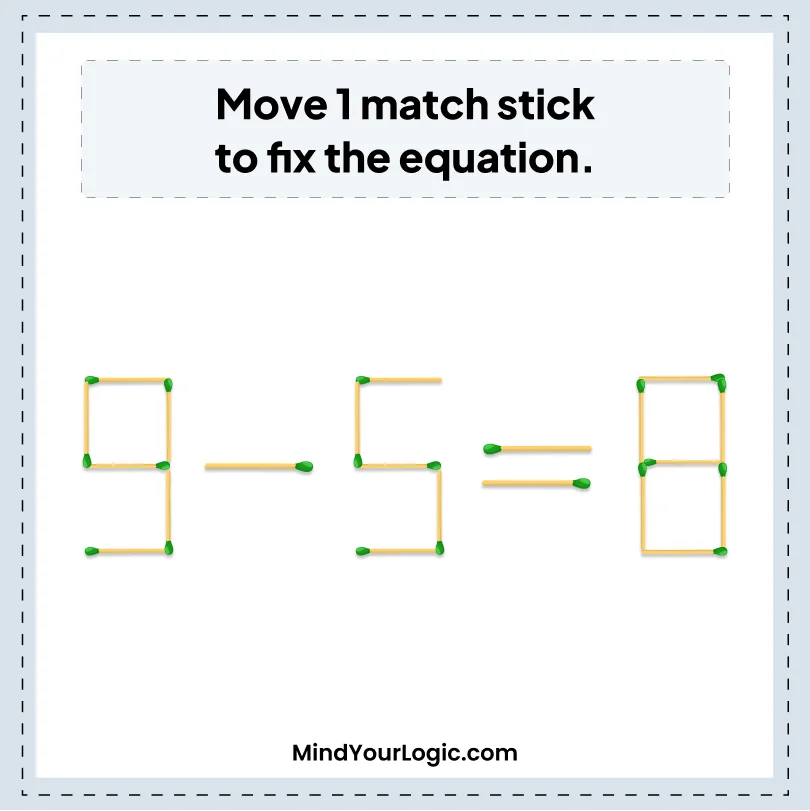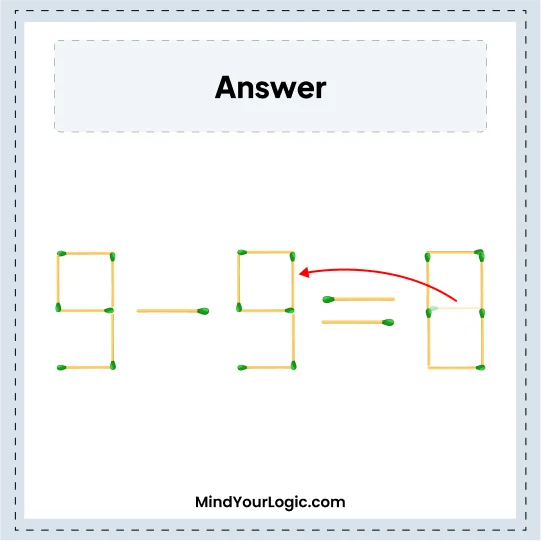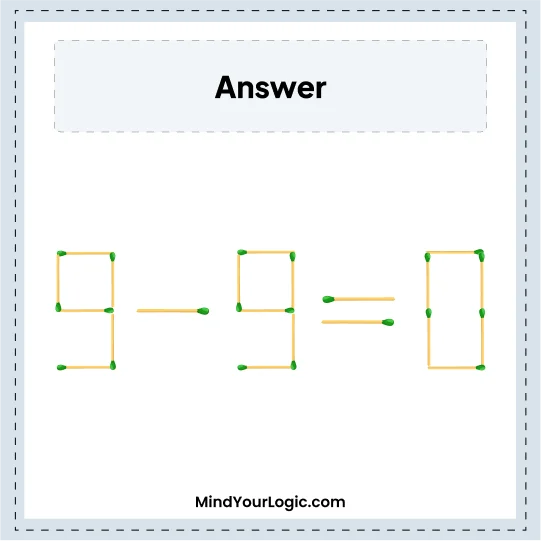# 9-5=8 - Matchstick Equation

###### 39.Matchstick Puzzles
`Move one matchstick and fix the equation in the correct order.`•

Explanation :

``` Move one matchstick from 8 and place it to 5.
So, 5 become 9 and 8 becomes 0.
The equation we get is 9 - 9 = 0.
```